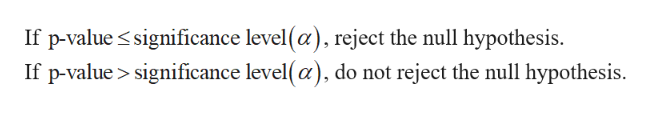# 1. True or False: If your alternative hypothesis is “not equal to”, then you will have a one-tailed test. 2. True or False: A p-value of 0.08 provides stronger evidence against the null hypothesis than a p-value of 0.10. 3. True or False: The t-distribution differs most from the normal distribution when we have a small sample size.

Question
1 views

1. True or False: If your alternative hypothesis is “not equal to”, then you will have a one-tailed test.

2. True or False: A p-value of 0.08 provides stronger evidence against the null hypothesis than a p-value of 0.10.

3. True or False: The t-distribution differs most from the normal distribution when we have a small sample size.

check_circle

Step 1

1.

If the alternative hypothesis states any direction (< or >), then the test is one-tailed test.

If the alternative hypothesis does not state any direction (≠), then the test is two-tailed test.

Here, the alternative hypothesis does not state any dir...help_outlineImage TranscriptioncloseIf p-value significance level(a), reject the null hypothesis If p-value> significance level(a), do not reject the null hypothesis fullscreen

### Want to see the full answer?

See Solution

#### Want to see this answer and more?

Solutions are written by subject experts who are available 24/7. Questions are typically answered within 1 hour.*

See Solution
*Response times may vary by subject and question.
Tagged in

### Statistics